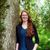# QlikView App Dev

Discussion Board for collaboration related to QlikView App Development.

Announcements
Join this live chat April 6, 10AM EST - QlikView to Qlik Sense REGISTER
cancel
Showing results for
Search instead for
Did you mean:Not applicable

## How do I script this?

I'm looking to create two expressions in a line chart:

If(severity = 'Critical' AND closed_date <> ' ')

If(severity = 'High' AND closed_date <> ' ')

if severity equals critical/high and the closed date is not empty

Thank you

1 Solution

Accepted SolutionsMVP

Create a flag in the script for empty closed date

LOAD If(Len(Trim(closed_date)) = 0, 0, 1) as Flag_Closed_Date

Sum({<Flag_Closed_Date = {1}, severity = {'Critical'}>}Measure)

Sum({<Flag_Closed_Date = {1}, severity = {'High'}>}Measure)

UPDATE: Just realized with Nicole's response that you want to exclude null here... I have updated the above, but you can also use this (in addition to Nicole's provided option)

Sum({<closed_date= {"=Len(Trim(closed_date)) > 0"}, severity = {'Critical'}>}Measure)

Sum({<closed_date= {"=Len(Trim(closed_date)) > 0"}, severity = {'High'}>}Measure)

9 RepliesMVP

Create a flag in the script for empty closed date

LOAD If(Len(Trim(closed_date)) = 0, 0, 1) as Flag_Closed_Date

Sum({<Flag_Closed_Date = {1}, severity = {'Critical'}>}Measure)

Sum({<Flag_Closed_Date = {1}, severity = {'High'}>}Measure)

UPDATE: Just realized with Nicole's response that you want to exclude null here... I have updated the above, but you can also use this (in addition to Nicole's provided option)

Sum({<closed_date= {"=Len(Trim(closed_date)) > 0"}, severity = {'Critical'}>}Measure)

Sum({<closed_date= {"=Len(Trim(closed_date)) > 0"}, severity = {'High'}>}Measure)MVP

Another option besides stalwar1's that will work as long as QlikView already knows that your closed_date is a date:

Sum({<severity = {'Critical'}, closed_date={'>0'}>}Measure)

Sum({<severity = {'High'}, closed_date={'>0'}>}Measure)Not applicable
Author

Hm, measure doesn't seem to be working for me.Champion III

What they meant by Measure is that your Fieldname you use to sum. Like

Sum({< ..... >} YourFieldName).Not applicable
Author

This is what i'm getting when using MonthYear as my measure. High is showing, but critical is not, any ideas?MVP

Convert this chart into a straight table and see if Critical shows up with any value or notNot applicable
Author

Straight table shows no values but a list box does. see picNot applicable
Author

Sum({<closed_date= {"=Len(Trim(closed_date)) > 0"}, severity = {'Critical'}>}Measure)

changed sum to count and this worked, thank you!MVP

Great